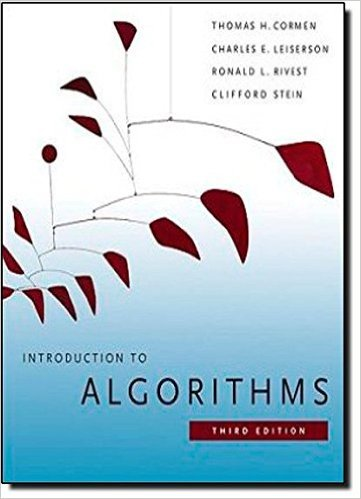×
×

# Searching an unsorted array This problem examines threeISBN: 9780262033848 130

## Solution for problem 5-2 Chapter 5

Introduction to Algorithms | 3rd Edition

• Textbook Solutions
• 2901 Step-by-step solutions solved by professors and subject experts
• Get 24/7 help from StudySoup virtual teaching assistantsIntroduction to Algorithms | 3rd Edition

4 5 1 409 Reviews
31
3
Problem 5-2

Searching an unsorted array This problem examines three algorithms for searching for a value x in an unsorted array A consisting of n elements. Consider the following randomized strategy: pick a random index i into A. If Ai D x, then we terminate; otherwise, we continue the search by picking a new random index into A. We continue picking random indices into A until we find an index j such that Aj D x or until we have checked every element of A. Note that we pick from the whole set of indices each time, so that we may examine a given element more than once. a. Write pseudocode for a procedure RANDOM-SEARCH to implement the strategy above. Be sure that your algorithm terminates when all indices into A have been picked. 144 Chapter 5 Probabilistic Analysis and Randomized Algorithms b. Suppose that there is exactly one index i such that Ai D x. What is the expected number of indices into A that we must pick before we find x and RANDOM-SEARCH terminates? c. Generalizing your solution to part (b), suppose that there are k 1 indices i such that Ai D x. What is the expected number of indices into A that we must pick before we find x and RANDOM-SEARCH terminates? Your answer should be a function of n and k. d. Suppose that there are no indices i such that Ai D x. What is the expected number of indices into A that we must pick before we have checked all elements of A and RANDOM-SEARCH terminates? Now consider a deterministic linear search algorithm, which we refer to as DETERMINISTIC-SEARCH. Specifically, the algorithm searches A for x in order, considering A1; A2; A3; : : : ; An until either it finds Ai D x or it reaches the end of the array. Assume that all possible permutations of the input array are equally likely. e. Suppose that there is exactly one index i such that Ai D x. What is the average-case running time of DETERMINISTIC-SEARCH? What is the worstcase running time of DETERMINISTIC-SEARCH? f. Generalizing your solution to part (e), suppose that there are k 1 indices i such that Ai D x. What is the average-case running time of DETERMINISTICSEARCH? What is the worst-case running time of DETERMINISTIC-SEARCH? Your answer should be a function of n and k. g. Suppose that there are no indices i such that Ai D x. What is the average-case running time of DETERMINISTIC-SEARCH? What is the worst-case running time of DETERMINISTIC-SEARCH? Finally, consider a randomized algorithm SCRAMBLE-SEARCH that works by first randomly permuting the input array and then running the deterministic linear search given above on the resulting permuted array. h. Letting k be the number of indices i such that Ai D x, give the worst-case and expected running times of SCRAMBLE-SEARCH for the cases in which k D 0 and k D 1. Generalize your solution to handle the case in which k 1. i. Which of the three searching algorithms would you use? Explain your answer.

Step-by-Step Solution:
Step 1 of 3
Step 2 of 3

Step 3 of 3

##### ISBN: 9780262033848

Unlock Textbook Solution